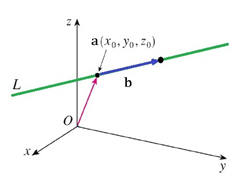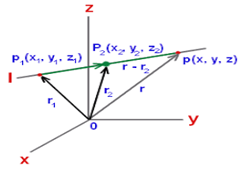#### The line passing through the pointscrosses the-plane at the pointThen Option 1)Option 2)Option 3)Option 4)As we learnt in

Cartesian eqution of a line -

The equation of a line passing through two pointsand parallel to vector having direction ratios asis given byThe equation of a line passing through two pointsis given by- whereinEquation of line isIf it crosses y-z plane, x=0Option 1)Incorrect Option

Option 2)Incorrect Option

Option 3)Incorrect Option

Option 4)Correct Option

#### divya.saini